## The Ideal Gas LawTemperature, volume, and pressure of a gas in ideal-gas-law action.

(Source)

We just learned that matter expands when heated and contracts when cooled. We also learned that fairly simple formulas calculate how much materials will expand or contract. Unfortunately these formulas don't work for gases. The situation's kind of non-ideal, doncha think? But we digress.

ΔV = βViΔT doesn't apply to gases, because gases expand a lot more than solids. More importantly, the volume and temperature of gases are affected by changes in pressure much more than the volume and temperature of solids and liquids.

Imagine, for example, a balloon. As we blow up the balloon, a gas (air) fills it and becomes trapped in the balloon. As more air shuffles into the balloon, the pressure in the balloon (and on the gas) increases. If we put 10 breaths of air into the balloon, it's pretty floppy. Another 50 breaths and the balloon is no longer squishy. Ten more breaths and—kaboom!—it pops. The air is trapped in the balloon, so adding more air increases the pressure on the balloon's elastic walls, and it's eventually so STRONK that the balloon breaks.

Here's a party trick for you: we can do the same thing by putting 50 breaths of air into the balloon and then heating it. As we heat the balloon, all of the air molecules start bouncing around faster and faster, taking up more and more space. It's essentially like adding more air. Eventually the molecules move so fast and take up so much space that they create just as much pressure on the balloon as those extra 10 breaths of air it took to break the balloon before, and—kaboom again!—the balloon pops.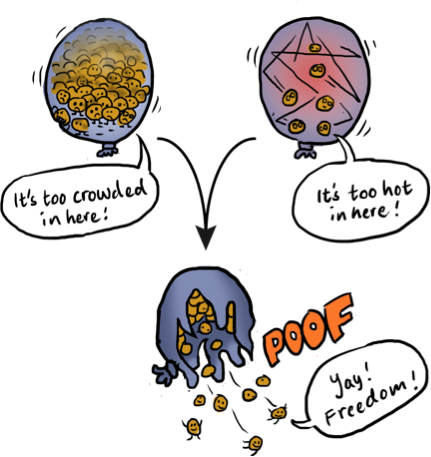The behavior of the air in a balloon can't be described by ΔV = β Vi ΔT. As we increase the temperature of the gas, the pressure exerted on the gas by the balloon increases, and this pressure affects ΔV, the change in volume, as well.

We need a new equation—better, stronger, faster—to explain how temperature affects the volume and pressure of gases. To find this mythic equation, we need to first talk about an important law of physics, Boyle's Law.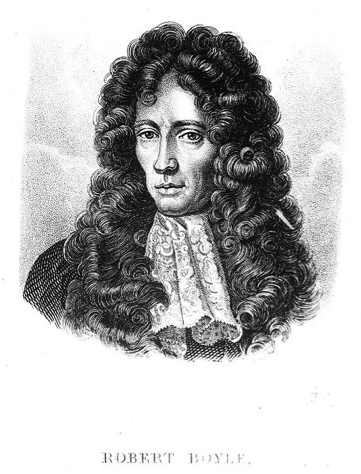Nice hair, there, Bob. You could be the ideal gas law lawyer.

(Source)

In the 1600s, Robert Boyle discovered that if the temperature of a gas is constant, then the product of the pressure and the volume of a gas is also a constant. Put a bit more mathily (but not too much), PV = constant, where P is pressure and V is volume.

What this says is that, if we have a certain volume of gas and the temperature doesn't change, then increasing the volume of the gas causes the pressure to decrease (or the other way around).

You've probably seen the issue already—the temperature isn't always constant. The white whale of an equation we're after will describe how pressure, volume, and the temperature of gases are all related to one another. If we change one, it will affect the others as well.

We can pop a balloon by squeezing it and changing the volume. We can also pop the balloon by increasing the temperature of the air inside. At a higher temperature the air molecules move around faster, the volume of air increases, the pressure on the balloon increases, and it pops. Boom.

In our earlier balloon example, we were talking about 10 breaths versus 50 breaths of air. The amount of air, or gas, is clearly important too. If we only put 10 breaths of air in a balloon we would have to squeeze it a lot harder, adding much more pressure, to pop it than if we had put 50 breaths in it.

So in addition to temperature, volume, and pressure of the gas, the amount of gas really matters too. When we include the amount of gas in our equation, we finally arrive at the ideal gas law. Take a look at it in all its glory.

PV = nRT

When scoping out this equation, P is pressure, V is volume, T is temperature, n is the number of moles of gas, and R is the universal gas constant.

Let's look a little closer at the nR part of this equation. It stands for the amount of gas. It tells you how many molecules of air we put into the balloon. R is a the universal gas constant, a proportion shared by all gases equal to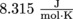.

What about this n thingy? Well, first of all, it's given in units called moles. This is the dreaded part where everyone wishes we could just talk about Mexican food, Cindy Crawford, and espionage, but in physics, chemistry, and biology a mole is a unit of measurement.

### Trillions of Molecules, Covered in Moles

A mole is a unit of measurement that tells us how many molecules of a particular substance are present. More specifically, a mole is 6.022 × 1023 molecules.

Molecules of what, you ask? Of anything: sugar, iron, cherry cola. Anything.

A mole of hydrogen contains 6.022 × 1023 molecules of hydrogen. A mole of oxygen contains 6.022 × 1023 molecules of oxygen. A mole of elephants? You guessed it: 6.022 × 1023 elephants. This number, 6.022 × 1023, even has a special name—Avogadro's number, NA.

To get an idea of just how mind-bogglingly huge that number is, bend your ear and listen to this song. The answer is, really mind-boggingly huge.

Okay, so a mole is 6.022 × 1023 molecules. How on Earth did anyone come up with such a definition? It turns out, some poor chap counted the number of molecules in 12 g of carbon and found that there are Avogadro's number, NA = 6.022 × 1023, molecules in 12 g of carbon, and then defined that quantity as a mole.

By definition, there are 6.022 × 1023 molecules in a mole of anything. But that doesn't mean that a mole of everything weighs the same. A mole of carbon-12 weighs 12 g. That same number, 6.022 × 1023, of molecules of oxygen, however, weighs 16 g. A mole of elephants? Well, multiply the weight of an average elephant, 5000 kg, by 6.022 × 1023. Heavy.

The fact that a mole of any substance contains 6.022 × 1023 molecules allows us to rewrite the ideal gas law in terms of the number of molecules of gas, N, instead of the number of moles of gas.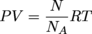This can be simplified again, because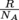is a constant (since R and NA are the same for any gas). This value,, is called Boltzmann's constant, kB, which is equal to the following.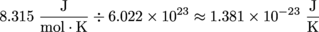And, at long last, the ideal gas law becomes PV = NkBT.

Wanna know a secret? We don't often use the ideal gas equation in its PV = nRT form. Many times, we're interested in changes to a gas within a closed system, like in our balloon examples. For these examples, nR is a constant, because the amount of gas remains the same. In other words: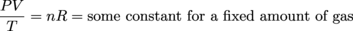When we compress the air in a balloon, we don't (i.e., can't) change the number of moles or the universal gas constant. Only a full-fledged Physics Wizard could do that, and we can't find our wand. For these experiments, we instead deal with pressure, temperature, and volume at two different points in time.

Because we assume the balloon is a closed system (so no heat, pressure, or volume can escape to the environment outside of the balloon) we have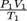before compression and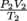after compression. In a closed systems, these proportions will be equivalent, such that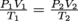.

This form of the ideal gas equation allows us to calculate one of these values when we know all of the others. Say, if we know all of the starting variables, and we push down on the balloon with a known pressure and temperature, then we can figure out what the volume will be. In a laboratory setting, these values are measured instead of "given".

In a literary setting, we'd give these values a close reading, and maybe engage in a little literary criticism with them. You probably shouldn't take the ideal gas law into an English class, though; they might just faint away.

### Common Mistakes

Make a note of the units for the universal gas constant, R. They are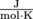. When we use the ideal gas law, all temperatures need to be in Kelvin (unit K).